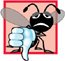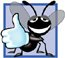# Examples Using the for Statement

The following examples show methods of varying the control variable in a for statement. In each case, we write the appropriate for statement header. Note the change in the relational operator for loops that decrement the control variable.

1. Vary the control variable from 1 to 100 in increments of 1.

```for ( int i = 1; i <= 100; i++ )
```
2. Vary the control variable from 100 down to 1 in increments of -1 (that is, decrements of 1).

```for ( int i = 100; i >= 1; i-- )
```
3. Vary the control variable from 7 to 77 in steps of 7.

```for ( int i = 7; i <= 77; i += 7 )
```
4. Vary the control variable from 20 down to 2 in steps of -2.

```for ( int i = 20; i >= 2; i -= 2 )
```
5. Vary the control variable over the following sequence of values: 2, 5, 8, 11, 14, 17, 20.

```for ( int i = 2; i <= 20; i += 3 )
```
6. Vary the control variable over the following sequence of values: 99, 88, 77, 66, 55, 44, 33, 22, 11, 0.

```for ( int i = 99; i >= 0; i -= 11 )
```

Common Programming Error 5.6Not using the proper relational operator in the loop-continuation condition of a loop that counts downward (such as incorrectly using i <= 1 instead of i >= 1 in a loop counting down to 1) is usually a logic error that yields incorrect results when the program runs.

Application: Summing the Even Integers from 2 to 20

The next two examples provide simple applications of the for statement. The program of Fig. 5.5 uses a for statement to sum the even integers from 2 to 20. Each iteration of the loop (lines 1213) adds the current value of the control variable number to variable total.

Figure 5.5. Summing integers with the for statement.

``` 1 // Fig. 5.5: fig05_05.cpp
2 // Summing integers with the for statement.
3 #include
4 using std::cout;
5 using std::endl;
6
7 int main()
8 {
9 int total = 0; // initialize total
10
11 // total even integers from 2 through 20
12 for ( int number = 2; number <= 20; number += 2 )
13  total += number;
14
15 cout << "Sum is " << total << endl; // display results
16 return 0; // successful termination
17 } // end main
```

 ``` Sum is 110 ```

Note that the body of the for statement in Fig. 5.5 actually could be merged into the increment portion of the for header by using the comma operator as follows:

```for ( int number = 2; // initialization
number <= 20; // loop continuation condition
total += number, number += 2 ) // total and increment
; // empty body
```

Good Programming Practice 5.6Although statements preceding a for and statements in the body of a for often can be merged into the for header, doing so can make the program more difficult to read, maintain, modify and debug.

Good Programming Practice 5.7Limit the size of control statement headers to a single line, if possible.

Application: Compound Interest Calculations

The next example computes compound interest using a for statement. Consider the following problem statement:

A person invests \$1000.00 in a savings account yielding 5 percent interest. Assuming that all interest is left on deposit in the account, calculate and print the amount of money in the account at the end of each year for 10 years. Use the following formula for determining these amounts:

a = p ( 1 + r ) n

where

p is the original amount invested (i.e., the principal),

r is the annual interest rate,

n is the number of years and

a is the amount on deposit at the end of the nth year.

This problem involves a loop that performs the indicated calculation for each of the 10 years the money remains on deposit. The solution is shown in Fig. 5.6.

Figure 5.6. Compound interest calculations with for.

(This item is displayed on pages 195 - 196 in the print version)

``` 1 // Fig. 5.6: fig05_06.cpp
2 // Compound interest calculations with for.
3 #include
4 using std::cout;
5 using std::endl;
6 using std::fixed;
7
8 #include
9 using std::setw; // enables program to set a field width
10 using std::setprecision;
11
12 #include  // standard C++ math library
13 using std::pow; // enables program to use function pow
14
15 int main()
16 {
17 double amount; // amount on deposit at end of each year
18 double principal = 1000.0; // initial amount before interest
19 double rate = .05; // interest rate
20
22 cout << "Year" << setw( 21 ) << "Amount on deposit" << endl;
23
24 // set floating-point number format
25 cout << fixed << setprecision( 2 );
26
27 // calculate amount on deposit for each of ten years
28 for ( int year = 1; year <= 10; year++ )
29 {
30  // calculate new amount for specified year
31  amount = principal * pow( 1.0 + rate, year );
32
33  // display the year and the amount
34  cout << setw( 4 ) << year << setw( 21 ) << amount << endl;
35 } // end for
36
37 return 0; // indicate successful termination
38 } // end main
```

 ``` Year Amount on deposit 1 1050.00 2 1102.50 3 1157.63 4 1215.51 5 1276.28 6 1340.10 7 1407.10 8 1477.46 9 1551.33 10 1628.89 ```

The for statement (lines 2835) executes its body 10 times, varying a control variable from 1 to 10 in increments of 1. C++ does not include an exponentiation operator, so we use the standard library function pow (line 31) for this purpose. The function pow( x, y ) calculates the value of x raised to the yth power. In this example, the algebraic expression ( 1 + r) n is written as pow( 1.0 + rate, year ), where variable rate represents r and variable year represents n. Function pow takes two arguments of type double and returns a double value.

This program will not compile without including header file (line 12). Function pow requires two double arguments. Note that year is an integer. Header includes information that tells the compiler to convert the value of year to a temporary double representation before calling the function. This information is contained in pow's function prototype. Chapter 6 provides a summary of other math library functions.

Common Programming Error 5.7In general, forgetting to include the appropriate header file when using standard library functions (e.g., in a program that uses math library functions) is a compilation error.

A Caution about Using Type double for Monetary Amounts

Notice that lines 1719 declare the variables amount, principal and rate to be of type double. We have done this for simplicity because we are dealing with fractional parts of dollars, and we need a type that allows decimal points in its values. Unfortunately, this can cause trouble. Here is a simple explanation of what can go wrong when using float or double to represent dollar amounts (assuming setprecision( 2 ) is used to specify two digits of precision when printing): Two dollar amounts stored in the machine could be 14.234 (which prints as 14.23) and 18.673 (which prints as 18.67). When these amounts are added, they produce the internal sum 32.907, which prints as 32.91. Thus your printout could appear as

``` 14.23
+ 18.67
-------
32.91
```

but a person adding the individual numbers as printed would expect the sum 32.90! You have been warned!

Good Programming Practice 5.8Do not use variables of type float or double to perform monetary calculations. The imprecision of floating-point numbers can cause errors that result in incorrect monetary values. In the Exercises, we explore the use of integers to perform monetary calculations. [Note: Some third-party vendors sell C++ class libraries that perform precise monetary calculations. We include several URLs in Appendix I].

Using Stream Manipulators to Format Numeric Output

The output statement at line 25 before the for loop and the output statement at line 34 in the for loop combine to print the values of the variables year and amount with the formatting specified by the parameterized stream manipulators setprecision and setw and the nonparameterized stream manipulator fixed. The stream manipulator setw( 4 ) specifies that the next value output should appear in a field width of 4i.e., cout prints the value with at least 4 character positions. If the value to be output is less than 4 character positions wide, the value is right justified in the field by default. If the value to be output is more than 4 character positions wide, the field width is extended to accommodate the entire value. To indicate that values should be output left justified, simply output nonparameterized stream manipulator left (found in header ). Right justification can be restored by outputting nonparameterized stream manipulator right.

The other formatting in the output statements indicates that variable amount is printed as a fixed-point value with a decimal point (specified in line 25 with the stream manipulator fixed) right justified in a field of 21 character positions (specified in line 34 with setw( 21 )) and two digits of precision to the right of the decimal point (specified in line 25 with manipulator setprecision( 2 )). We applied the stream manipulators fixed and setprecision to the output stream (i.e., cout) before the for loop because these format settings remain in effect until they are changedsuch settings are called sticky settings. Thus, they do not need to be applied during each iteration of the loop. However, the field width specified with setw applies only to the next value output. We discuss C++'s powerful input/output formatting capabilities in detail in Chapter 15.

Note that the calculation 1.0 + rate, which appears as an argument to the pow function, is contained in the body of the for statement. In fact, this calculation produces the same result during each iteration of the loop, so repeating it is wastefulit should be performed once before the loop.

Performance Tip 5.1Avoid placing expressions whose values do not change inside loopsbut, even if you do, many of today's sophisticated optimizing compilers will automatically place such expressions outside the loops in the generated machine-language code.

Performance Tip 5.2Many compilers contain optimization features that improve the performance of the code you write, but it is still better to write good code from the start.

For fun, be sure to try our Peter Minuit problem in Exercise 5.29. This problem demonstrates the wonders of compound interest.C++ How to Program (5th Edition)
ISBN: 0131857576
EAN: 2147483647
Year: 2004
Pages: 627